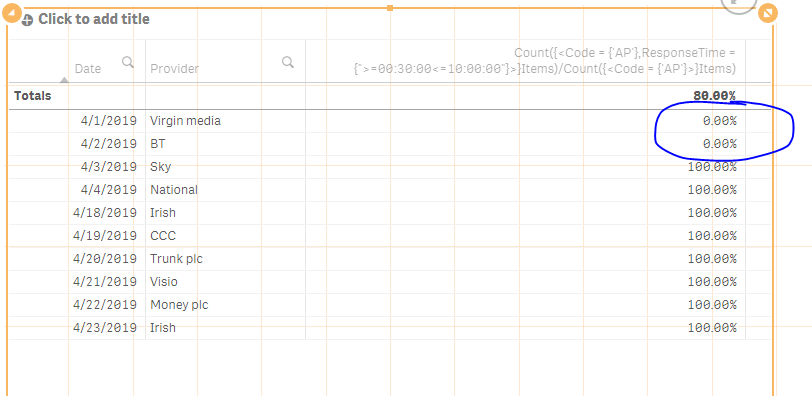# Qlik Sense App Development

Announcements
Qlik Analytics Tour 2020 Online. Begins August 10th. Register Today
cancel
Showing results for
Did you mean:
HighlightedContributor III

## Set analysis - Percentage Count

Please shed some light on below expression I’m trying to show the count of percentages only  if the percentage is < 50%

My expression is =Count({<Status-={'REJECTED'},Code ={'AP'} Items) / Count ({<Status-={'REJECTED'}>}Items)
The above expression gives me 40%  so my count is 1.

My Dimension= Date, Product
Expression is Count of  items if the above expression is <50% Items)

Expected Result:

Straight table

Date            Provider   Count of Expression(only <50%)

28/04/2019    002        1

27/04/2019    019        5

Labels (1)
• ### Qlik Sense Deployment

1 Solution

Accepted Solutions
HighlightedMVP

## Re: Set analysis - Percentage Count

So, for the sample data provided... this gives me 2

Sum(Aggr(

If(Count({<Code = {'AP'},ResponseTime = {">=00:30:00<=10:00:00"}>}Items)/Count({<Code = {'AP'}>}Items) < 0.50, 1, 0)

, Date, Provider))

Expression is different because the chart dimensions are different.... this expression is based on Provider being the second dimension....

8 Replies
HighlightedMVP

## Re: Set analysis - Percentage Count

May be this

Sum(Aggr(

If(Count({<Status-={'REJECTED'},Code ={'AP'} Items) / Count ({<Status-={'REJECTED'}>}Items) < 0.50, 1, 0)

, Date, Item))

HighlightedContributor III

## Re: Set analysis - Percentage Count

Hi Sunny, Sorry for the delay reply. I am getting the output of '0' normally i would expect numbers. is that something issue with my dimensions, (Date, Provider)..? on the above expression
HighlightedMVP

## Re: Set analysis - Percentage Count

Would you be able to share some sample data?

HighlightedContributor III

## Re: Set analysis - Percentage Count

I'm working on Hub, not sure how to share he qvf file. But i am sharing some sample data with the straight table screenshot Here : Straight table

Dimentions are Date, Provider

Expression: Count({< code={'AP'},ResponseTime={'>=00:30:00<=10:00:00'}>}Items)/Count({< code={'AP'}>}Items)

Want to show another expression would be count of any percentage which is <50 %

my dummy data shows 0% and 100% only. basically in this case i want to show the count of 0%'s because 0% is <50. please do me know if its clear.?

HighlightedMVP

## Re: Set analysis - Percentage Count

So, in this case, you would want a count of 2?HighlightedContributor III

## Re: Set analysis - Percentage Count

Yes Exactly..thats right I am looking to see the Count of 2, but your previous expression giving me '0' please suggest me the solution.

HighlightedMVP

## Re: Set analysis - Percentage Count

So, for the sample data provided... this gives me 2

Sum(Aggr(

If(Count({<Code = {'AP'},ResponseTime = {">=00:30:00<=10:00:00"}>}Items)/Count({<Code = {'AP'}>}Items) < 0.50, 1, 0)

, Date, Provider))

Expression is different because the chart dimensions are different.... this expression is based on Provider being the second dimension....

HighlightedContributor III

## Re: Set analysis - Percentage Count

you are star. thanks for the help. much appreciated.